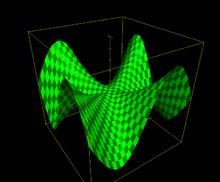# Symmetry of second derivatives

﻿
Symmetry of second derivatives

In mathematics, the symmetry of second derivatives (also called the equality of mixed partials) refers to the possibility of interchanging the order of taking partial derivatives of a function$f(x_{1},x_{2}, \dots ,x_{n})$

of n variables. If the partial derivative with respect to xi is denoted with a subscript i, then the symmetry is the assertion that the second-order partial derivatives fij satisfy the identity

fij = fji

so that they form an n × n symmetric matrix. This is sometimes known as Young's theorem.

This property may also be considered as a condition for the function$f(x_{1},x_{2}, \dots ,x_{n})$ to be single-valued. Then it is called the Schwarz integrability condition. In physics, however, it is important for the understanding of many phenomena in nature to remove this restrictions and allow functions to violate the Schwarz integrability criterion, which makes them multivalued. The simplest example is the function$\arctan\; y/x$. At first one defines this with a cut in the complex (x,y)-plane running from 0 to infinity. The cut makes the function single-valued. In complex analysis, however, one thinks of this function as having several 'sheets' (forming a Riemann surface).

## Hessian matrix

This matrix of second-order partial derivatives of f is called the Hessian matrix of f. The entries in it off the main diagonal are the mixed derivatives; that is, successive partial derivatives with respect to different variables.

In most circumstances the Hessian matrix is symmetric. Mathematical analysis reveals that symmetry requires a hypothesis on f that goes further than simply stating the existence of the second derivatives at a particular point. Clairaut's theorem gives a sufficient condition on f for this to occur.

## Formal expressions of symmetry

In symbols, the symmetry says that, for example,$\frac {\partial}{\partial x} \left( \frac { \partial f }{ \partial y} \right) = \frac {\partial}{\partial y} \left( \frac { \partial f }{ \partial x} \right).$

This equality can also be written as$\partial_{xy} f = \partial_{yx} f.$

Alternatively, the symmetry can be written as an algebraic statement involving the differential operator Di which takes the partial derivative with respect to xi:

Di . Dj = Dj . Di.

From this relation it follows that the ring of differential operators with constant coefficients, generated by the Di, is commutative. But one should naturally specify some domain for these operators. It is easy to check the symmetry as applied to monomials, so that one can take polynomials in the xi as a domain. In fact smooth functions are possible.

## Clairaut's theorem

In mathematical analysis, Clairaut's theorem or Schwarz's theorem, named after Alexis Clairaut and Hermann Schwarz, states that if$f \colon \mathbb{R}^n \to \mathbb{R}$

has continuous second partial derivatives at any given point in$\mathbb{R}^n$, say,$(a_1, \dots, a_n),$ then for$1 \leq i,j \leq n,$$\frac{\partial^2 f}{\partial x_i\, \partial x_j}(a_1, \dots, a_n) = \frac{\partial^2 f}{\partial x_j\, \partial x_i}(a_1, \dots, a_n).\,\!$

In words, the partial derivations of this function are commutative at that point. One easy way to establish this theorem (in the case where n = 2, i = 1, and j = 2, which readily entails the result in general) is by applying Green's theorem to the gradient of f.

## Distribution theory formulation

The theory of distributions eliminates analytic problems with the symmetry. The derivative of any integrable function can be defined as a distribution. The use of integration by parts puts the symmetry question back onto the test functions[clarification needed], which are smooth and certainly satisfy the symmetry. In the sense of distributions, symmetry always holds.

Another approach, which defines the Fourier transform of a function, is to note that on such transforms partial derivatives become multiplication operators that commute much more obviously.

## Non-symmetry

In the worst case symmetry fails. Given two variables near (0, 0) and two limiting processes on

f(h,k) − f(h,0) − f(0,k) + f(0,0)

corresponding to making h → 0 first, and to making k → 0 first. These processes need not commute (see interchange of limiting operations): it can matter, looking at the first-order terms, which is applied first. This leads to the construction of pathological examples in which second derivatives are non-symmetric. Given that the derivatives as Schwartz distributions are symmetric, this kind of example belongs in the 'fine' theory of real analysis.f(x,y)

The following example displays non-symmetry. Note that it does not violate Clairaut's theorem since the derivatives are not continuous at (0,0)$f(x,y) = \begin{cases} \frac{xy(x^2 - y^2)}{x^2+y^2} & \mbox{ for } (x, y) \ne (0, 0)\\ 0 & \mbox{ for } (x, y) = (0, 0). \end{cases}$

The mixed partial derivatives of f exist and are continuous everywhere except at (0,0). Moreover$\frac {\partial}{\partial x} \left( \frac { \partial f }{ \partial y} \right) \ne \frac {\partial}{\partial y} \left( \frac { \partial f }{ \partial x} \right)$

at (0,0).

## In Lie theory

Consider the first-order differential operators Di to be infinitesimal operators on Euclidean space. That is, Di in a sense generates the one-parameter group of translations parallel to the xi-axis. These groups commute with each other, and therefore the infinitesimal generators do also; the Lie bracket

[Di, Dj] = 0

is this property's reflection. In other words, the Lie derivative of one coordinate with respect to another is zero.

Wikimedia Foundation. 2010.